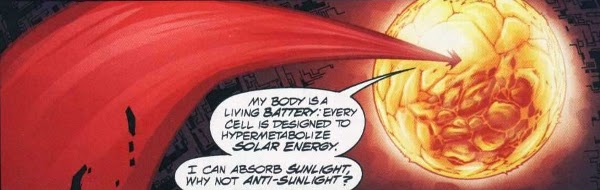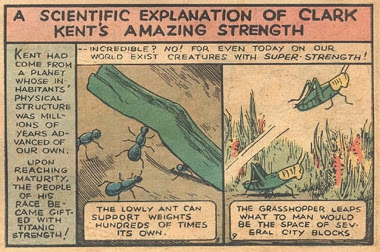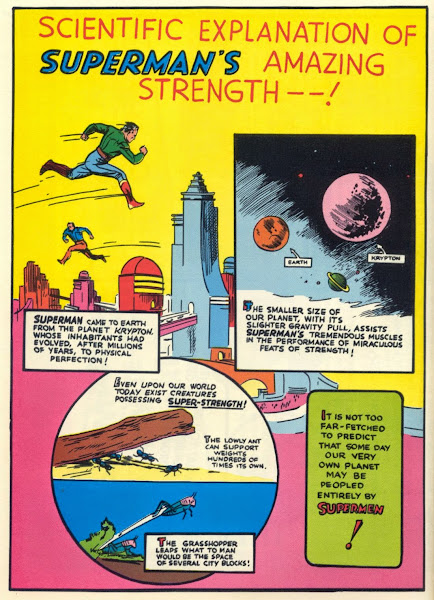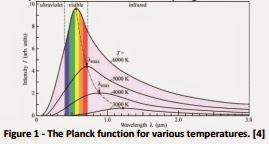### The solar efficiency of Superman

by @ulaulaman http://t.co/WGbVdfv0nk about #Superman #physics and #solar #energy
In the last saga of the JLA by Grant Morrison, World War III, Superman, leaping against the bomb inside Mageddon says:The way in which Superman gets the powers, or the way in which them is explained, however, is changed over time. Following Action Comics #1, the debut of the character, Jerry Siegel, combining genetics and evolution, says that on his planet of origin
the physical structure of the inhabitants had advanced millions of years compared to ours. Reaching maturity, people of that race earned a titanic force!In Superman #1, however, Siegel focuses attention on the different gravity between Earth and Krypton, with the latter with a greater radius than aour planet and therefore with a greater severity. Such a claim is also in Ports of Call by Jack Vance. In order to verify it, we must start from the definition of the density: $\rho = \frac{M}{V}$ where $M$ is the mass, $V$ the volume of the object, or, in our case, of the planet.
Consider, therefore, the formula of the gravitational interaction between two bodies, the planet $M$ and a satellite $m$. So, using the previous formula, we can express the planet's mass in the following way: $M = \rho V$ and so the attraction in the following way: $F = m \frac{G}{r^2} \rho V = mg$ Now we find the gravity: $g = G \frac{\rho V}{r^2}$ and using the volume of a sphere $V = \frac{4}{3} \pi r^3$ we finally obtain the gravity depending on density and radius: $g = \frac{4}{3} \pi G \rho r$ We find, therefore, that is an expression that depends on the radius of the planet, and its density. This means that, in the presence of a rocky planet with a density therefore not too different from that of the Earth, Jack Vance's and Jerry Siegel's statement is essentially correct.Back to Superman: only with the silver age was introduced the concept of the dependence of Superman's powers by the solar energy, in this way transforming the Man of Steel in a real solar battery: this point has been tested in a short article, The solar cell efficency of Superman, released at the end of October 2013 on the Journal of Physics Special Topics. The questions explored in the article are basically two: if the differences between the Sun and Rao, the red star of Krypton, can justify a higher energy output by our star and, more importantly, to understand the efficiency of Superman as a solar panel.The first question is fairly simple and follows a simple graph on the issue of the various types of stars, which shows that the appropriate conditions, a yellow star can emit a higher energy than a red star. This, however, also suggests another detail: In many attacks carried out against Superman, are used radiation type red star (see, for example, in Gog). The graph suggests that, also in this case, the red radiations must have the particular physical properties to be truly lethal against Superman.
The question of efficiency, however, is that on which researchers spend more calculations: evaluating the hero as an average human being, after half a page of calculations, they arrive at a very high value of efficiency: 656000%, a value that is definitely very high not only compared to the best efficiency of solar panels terrestrial (a little less than 45%), but also compared to the efficiency of any ideal thermodynamic machine (100%), which would also results in a rapid depletion of energy at its disposal.
Perhaps DC Comics would resume using the differences in gravitational or some substantial genetic difference to explain the superpowers of Superman.
via Oil Price